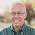Thursday, February 16, 2017

A nice Bayes theorem problem: medical testing

On these previous post about my favorite Bayes theorem problems, I got the following comment from a reader named Riya:

I have a question. Exactly 1/5th of the people in a town have Beaver Fever . There are two tests for Beaver Fever, TEST1 and TEST2. When a person goes to a doctor to test for Beaver Fever, with probability 2/3 the doctor conducts TEST1 on him and with probability 1/3 the doctor conducts TEST2 on him. When TEST1 is done on a person, the outcome is as follows: If the person has the disease, the result is positive with probability 3/4. If the person does not have the disease, the result is positive with probability 1/4. When TEST2 is done on a person, the outcome is as follows: If the person has the disease, the result is positive with probability 1. If the person does not have the disease, the result is positive with probability 1/2. A person is picked uniformly at random from the town and is sent to a doctor to test for Beaver Fever. The result comes out positive. What is the probability that the person has the disease?

I think this is an excellent question, so I am passing it along to the readers of this blog.  One suggestion: you might want to use my world famous Bayesian update worksheet.

Hint: This question is similar to one I wrote about last year.  In that article, I started with a problem that was underspecified; it took a while for me to realize that there were several ways to formulate the problem, with different answers.

Fortunately, the problem posed by Riya is completely specified; it is an example of what I called Scenario A, where there are two tests with different properties, and we don't know which test was used.

There are several ways to proceed, but I recommend writing four hypotheses that specify the test and the status of the patient:

TEST1 and sick
TEST1 and not sick
TEST2 and sick
TEST2 and not sick

For each of these hypotheses, it is straightforward to compute the prior probability and the likelihood of a positive test.  From there, it's just arithmetic.

Here's what it looks like using my world famous Bayesian update worksheet:

(Now with more smudges because I had an arithmetic error the first time.  Thanks, Ben Torvaney, for pointing it out.)

After the update, the total probability that the patient is sick is 10/26 or about 38%.  That's up from the prior, which was 1/5 or 20%.  So the positive test is evidence that the patient is sick, but it is not very strong evidence.

Interestingly, the total posterior probability of TEST2 is 12/26 or about 46%.  That's up from the prior, which was 33%.  So the positive test provides some evidence that TEST2 was used.

1.Hey thanks Mr. Allen for taking up the question.I would just like to mention that I came across this question from the MIT OCW final exam for computer science.
I have thought of two approaches and of course they're giving different answers, although I'm a little biased towards one of the approaches. I would be really glad if I could finally understand what's happening in this question.

1.Thanks for letting me know about the source of the problem. If you have a link, I'll include it in the article.

2.I enjoyed the problem, but I got a different answer. I thought the prior for TEST1 was 2/3.

1.I have corrected that error. Thanks!

3.This comment has been removed by a blog administrator.

4.I have a question though. I mentioned that this question was taken from the MIT 2006 OCW final exam for computer science. The solution was also available on the internet. Even though they have mentioned the answer to be 5/13 I.e. 10/26 but there's some calculation error in their method. The correct answer according to their method is 25/63. But I really don't understand why they did the question that way. I'm adding the links to both the question and the answer here. It's the fourth question.
1.